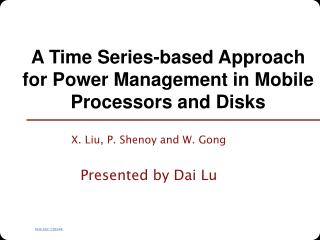DownloadDownload PresentationA Time Series-based Approach for Power Management in Mobile Processors and Disks

# A Time Series-based Approach for Power Management in Mobile Processors and Disks

Download Presentation## A Time Series-based Approach for Power Management in Mobile Processors and Disks

- - - - - - - - - - - - - - - - - - - - - - - - - - - E N D - - - - - - - - - - - - - - - - - - - - - - - - - - -
##### Presentation Transcript

1. A Time Series-based Approach for Power Management in Mobile Processors and Disks X. Liu, P. Shenoy and W. Gong Presented by Dai Lu

2. Contents • Introduction • Time Series based Power Management • Utilization Measurement • Prediction Model • Speed Setting Strategy • Implementation • Evaluation • Summary

3. Introduction • Multimedia applications prevalent on mobile devices • 3G/4G wireless network • Devices more and more powerful • Samsung SPH-V5400 hand phone is equipped with a 1.5 GB micro drive • Energy is a scarce resource

4. Previous Work • CPU DVFS: Dynamic Voltage and Frequency Scaling • Infer task periodicity by work-tracking heuristic • Assume implicit deadlines for interactive applications • Only periodic applications; assumes applications tell OS their periods and work amount • Disk DRPM: Dynamic Rotations Per Minute • Monitor disk request queue length • On-disk cache impact not considered

5. Why DRPM? • Power- RPM relation • Ke: spindle motor voltage • R: motor resistance • ω: angular velocity • Similar to DVS for processors (P~fV2)

6. Contents • Introduction • Time Series based Power Management • Utilization Measurement • Prediction Model • Speed Setting Strategy • Implementation • Evaluation • Summary

7. New Work • Low overhead • Prediction with simple statistical model in time series analysis • Processor + disk • TS-DVFS + TS-DRPM • Different CPU scaling factor for different tasks • Enable coexistence of MM and non-MM applications

8. TS-PM enabled OS kernel

9. Prediction Model • Box-Jenkins model in time series analysis • Assume a stationary process • Statistical properties (mean, variance) are essentially constant through time. • Firs-order autoregressive process (AR(1)) predictor • ũt = Φ1ũt-1+at • Φ1: Correlation coefficient • at:Error/ random shock • Sample Autocorrelation Function (SAC)

10. Estimated demand: Estimated mean: Estimated constant( SAC): Prediction Model Cont. • TS-DVFS: one AR(1) for every task • TS-DRPM: a single AR(1)

11. Measuring utilization • CPU e: full-speed execution time q: time quantum allocated to the task • Disk • r: response time • s: scaling factor

12. Speed Setting Strategy • TS-DVFS • Two level CPU setting • Interval T • Subinterval within T

13. Speed Setting Strategy • TS-DRPM • Performance slow-down • Pdiff[i] = a(1-h) × T × Rdiff[i] • Estimated utilization • ûi = û + Pdiff[i]/ T h: hit rate a: arrival rate Rdiff: rotational latency difference • Choose the lowest RPM level satisfying • (ûi- ûmax) / ûmax ≤ threshold

14. Contents • Introduction • Time Series based Power Management • Utilization Measurement • Prediction Model • Speed Setting Strategy • Implementation • Evaluation • Summary

15. Implementation • CPU • 300-677 MHz, Transmeta • Divide into 5 steps • Mapping scaling factor to frequency level • Disk • 3000-5400 RPM • Divide into 5 steps • Assumed power consumption level • Trace driven simulation with DiskSim

16. Frequency and RPM Mapping

17. Contents • Introduction • Time Series based Power Management • Utilization Measurement • Prediction Model • Speed Setting Strategy • Implementation • Evaluation • Summary

18. TS-DVFS Up to 38.6% energy saving against LongRun

19. TS-DRPM Up to 20.3% saving against TPMperf (oracle)

20. Summary • Time series statistical model • TS-DVFS • TS-DRPM • Comments • General PM, no QoS measurement like deadline miss rate • Multiple rotational speed disk not commercially available • Increase the accuracy of profiling disk access patterns. • “Hit if response time < τ, otherwise miss.”

21. References • Chameleon: Application Controlled Power Management with Performance Isolation, X. Liu and P. Shenoy, Technical report 04-26, Department of Computer Science, University of Massachusetts • Forecasting and time series: an applied approach 3rd ed, Bowerman and O’Connell, Duxbury, 1993 • Reducing disk power consumption in servers with DRPM, S. Gurumurthi, A. Sivasubramaniam and H. Franke, IEEE Computer, Dec 2003